## 7.3 – Factoring Trinomials

### Learning Objectives

• (7.3.1) – Factoring out the greatest common factor
• Identify the greatest common factor of a polynomial
• Factor the greatest common factor out of a polynomial
• Factoring out a greatest common factor that is a binomial
• (7.3.2) – Factor a Trinomial with Leading Coefficient = 1
• Use a method to factor a trinomial with a leading coefficient of 1
• Recognize when a trinomial cannot be factored (prime)
• (7.3.3) – Factor a Trinomial with Leading Coefficient $\neq 1$
• Factor by trial and error
• Factor by Grouping

Factors are the building blocks of multiplication. They are the numbers that you can multiply together to produce another number: 2 and 10 are factors of 20, as are 4, 5, 1, 20. To factor a number is to rewrite it as a product. $\displaystyle 20=4\cdot5� . In algebra, we use the word factor as both a noun – something being multiplied – and as a verb – the action of rewriting a sum or difference as a product. Factoring is very helpful in simplifying expressions and solving equations involving polynomials. # (7.3.1) – Factoring out the Greatest common factor The greatest common factor (GCF) of two numbers is the largest number that divides evenly into both numbers. For instance, [latex]4$ is the GCF of $16$ and $20$ because it is the largest number that divides evenly into both $16$ and $20$ The GCF of polynomials works the same way: $4x$ is the GCF of $16x$ and $20{x}^{2}$ because it is the largest polynomial that divides evenly into both $16x$ and $20{x}^{2}$.

When factoring a polynomial expression, our first step should be to check for a GCF. Look for the GCF of the coefficients, and then look for the GCF of the variables.

### Greatest Common Factor

The greatest common factor (GCF) of two or more expressions is the largest expression that is a factor of all the expressions (both the coefficient and the variable with the highest degree.)

### Example

Find the greatest common factor of $25b^{3}$ and $10b^{2}$.

The video that follows gives an example of finding the greatest common factor of two monomials with only one variable.

Sometimes you may encounter a polynomial with more than one variable, so it is important to check whether both variables are part of the GCF. In the next example we find the GCF of two terms which both contain two variables.

### Example

Find the greatest common factor of $81c^{3}d$ and $45c^{2}d^{2}$.

The video that follows shows another example of finding the greatest common factor of two monomials with more than one variable.

Now that you have practiced identifying the GCF of a term with one and two variables, we can apply this idea to factoring the GCF out of a polynomial. Notice that the instructions are now “Factor” instead of “Find the greatest common factor”.

To factor a polynomial, first identify the greatest common factor of the terms. You can then use the distributive property to rewrite the polynomial in a factored form. Recall that the distributive property of multiplication over addition states that a product of a number and a sum is the same as the sum of the products.

#### Distributive Property Forward and Backward

Forward: Product of a number and a sum: $a\left(b+c\right)=a\cdot{b}+a\cdot{c}$. You can say that “$a$ is being distributed over $b+c$.”

Backward: Sum of the products: $a\cdot{b}+a\cdot{c}=a\left(b+c\right)$. Here you can say that “$a$ is being factored out.”

We first learned that we could distribute a factor over a sum or difference, now we are learning that we can “undo” the distributive property with factoring.

### Example

Factor $25b^{3}+10b^{2}$.

The factored form of the polynomial $25b^{3}+10b^{2}$ is $(5b^{2})\left(5b+2\right)$. You can check this by doing the multiplication. $(5b^{2})\left(5b+2\right)=25b^{3}+10b^{2}$.

Note that if you do not factor the greatest common factor at first, you can continue factoring, rather than start all over.

For example:

$\begin{array}{l}25b^{3}+10b^{2}=5\left(5b^{3}+2b^{2}\right)\,\,\,\,\,\,\,\,\,\,\,\text{Factor out }5.\\\,\,\,\,\,\,\,\,\,\,\,\,\,\,\,\,\,\,\,\,\,\,\,\,\,\,\,\,\,=(5b^{2})\left(5b+2\right) \,\,\,\,\,\,\,\,\,\,\,\,\,\,\,\text{Factor out }b^{2}.\end{array}$

Notice that you arrive at the same simplified form whether you factor out the GCF immediately or if you pull out factors individually.

In the following video we show two more examples of how to find and factor the GCF from binomials.

We will show one last example of finding the GCF of a polynomial with several terms and two variables. No matter how large the polynomial, you can use the same technique described below to factor out it’s GCF.

### How To: Given a polynomial expression, factor out the greatest common factor.

1. Identify the GCF of the coefficients.
2. Identify the GCF of the variables.
3. Combine to find the GCF of the expression.
4. Determine what the GCF needs to be multiplied by to obtain each term in the expression.
5. Write the factored expression as the product of the GCF and the sum of the terms we need to multiply by.

### Example

Factor $6{x}^{3}{y}^{3}+45{x}^{2}{y}^{2}+21xy$.

In the following video you will see two more example of how to find and factor out the greatest common factor of a polynomial.

### Factoring out a GCF that is a binomial

Next we present two examples where we can factor out a binomial term from both expressions.

### ExAMPLE

Factor $3y(y+7)-4(y+7)$.

### ExAMPLE

Factor $y(x+3)+2(x+3)$.

# (7.3.2) – Factor a Trinomial with Leading Coefficient = 1

Trinomials are polynomials with three terms. We are going to show you a method for factoring a trinomial whose leading coefficient is 1.  Although we should always begin by looking for a GCF, pulling out the GCF is not the only way that trinomials can be factored. The trinomial ${x}^{2}+5x+6$ has a GCF of 1, but it can be written as the product of the factors $\left(x+2\right)$ and $\left(x+3\right)$.

Recall how to use the distributive property to multiply two binomials:

$\left(x+2\right)\left(x+3\right) = x^2+3x+2x+6=x^2+5x+6$

We can reverse the distributive property and return $x^2+5x+6\text{ to }\left(x+2\right)\left(x+3\right)$ by finding two numbers with a product of $6$ and a sum of $5$.

### Factoring a Trinomial with Leading Coefficient 1

In general, for a trinomial of the form ${x}^{2}+bx+c$ you can factor a trinomial with leading coefficient 1 by finding two numbers,$p$ and $q$ whose product is $c$, and whose sum is $b$. The trinomial will then factor into $(x+p)(x+q)$, with $pq=c$ and $p+q=b$.

Let’s put this idea to practice with the following example.

### Example

Factor ${x}^{2}+2x - 15$.

In the following video we present two more examples of factoring a trinomial with a leading coefficient of 1.

To summarize our process consider these steps:

### How To: Given a trinomial in the form ${x}^{2}+bx+c$, factor it.

1. List factors of $c$.
2. Find $p$ and $q$, a pair of factors of $c$ with a sum of $b$.
3. Write the factored expression $\left(x+p\right)\left(x+q\right)$.

We will now show an example where the trinomial has a negative c term. Pay attention to the signs of the numbers that are considered for p and q.

In our next example, we show that when c is negative, either p or q will be negative.

### Example

Factor $x^{2}+x-12$.

Which property of multiplication can be used to describe why $\left(x+4\right)\left(x-3\right) =\left(x-3\right)\left(x+4\right)$. Use the textbox below to write down your ideas before you look at the answer.

In our next example we will show how to factor a trinomial whose $b$ term is negative.

### Example

Factor ${x}^{2}-7x+6$.

In the next example, we factor a trinomial with two variable, but the leading coefficient is still 1.

### ExAMPLE

Factor: $x^2-8xy-9y^2$

### Recognize when a trinomial cannot be factored (prime)

Can every trinomial be factored as a product of binomials?

Mathematicians often use a counter example to prove or disprove a question. A counter example means you provide an example where a proposed rule or definition is not true. Can you create a trinomial with leading coefficient 1 that cannot be factored as a product of binomials?

Use the textbox below to write your ideas.

### Definition

A polynomial is prime if it cannot be factored using integer coefficients. A polynomial is factored completely when it is written as the product of prime polynomials.

For example, the polynomial $x^2+x+1$ is a prime.

# (7.3.3) – Factor a Trinomial with Leading Coefficient $\neq 1$

### Factor by Trial and Error

What happens when the leading coefficient is not 1 and there is no GCF? There are several methods that can be used to factor these trinomials. First we will use the Trial and Error method.

Let’s factor the trinomial using Trial and Error: $3y^2+22y+7$.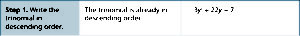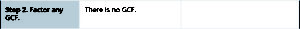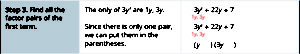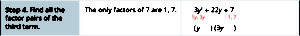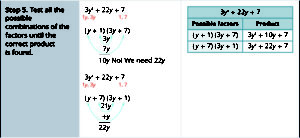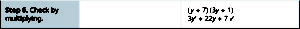Try another example, this time with a negative leading coefficient: (Hint: try factoring out $-1$ out first.)

### Factor by Grouping

Trinomials with leading coefficients other than 1 are slightly more complicated to factor. For these trinomials, we can factor by grouping by dividing the x term into the sum of two terms, factoring each portion of the expression separately, and then factoring out the GCF of the entire expression. The trinomial $2{x}^{2}+5x+3$ can be rewritten as $\left(2x+3\right)\left(x+1\right)$ using this process. We begin by rewriting the original expression as $2{x}^{2}+2x+3x+3$ and then factor each portion of the expression to obtain $2x\left(x+1\right)+3\left(x+1\right)$. We then pull out the GCF of $\left(x+1\right)$ to find the factored expression.

The first step in this process is to figure out what two numbers to use to re-write the x term as the sum of two new terms. Making a table to keep track of your work is helpful. We are looking for two numbers with a product of $2\cdot3=6$ and a sum of $5$

Factors of $2\cdot3=6$ Sum of Factors
$1,6$ $7$
$-1,-6$ $-7$
$2,3$ $5$
$-2,-3$ $-5$

The pair $p=2,\text{ and }q=3$ will give the correct x term, so we will rewrite it using the new factors:

$2{x}^{2}+5x+3=2x^2+2x+3x+3$

Now we can group the polynomial into two binomials.

$2x^2+2x+3x+3=(2x^2+2)+(3x+3)$

Identify the GCF of each binomial.

2x is the GCF of $(2x^2+2)$ and 3 is the GCF of $(3x+3)$, use this to rewrite the polynomial:

$(2x^2+2x)+(3x+3)=2x(x+1)+3(x+1)$

Note how we leave the signs in the binomials and the addition that joins them, be careful with signs when you factor out the GCF. The GCF of our new polynomial is $(x+1)$, we factor this out as well:

$2x(x+1)+3(x+1)=(x+1)(2x+3)$.

Sometimes it helps visually to write the polynomial this way $(x+1)2x+(x+1)3$ before you factor out the GCF. This is purely a matter of preference, multiplication is commutative, so order doesn’t matter.

### A General Note: Factor by Grouping

To factor a trinomial in the form $a{x}^{2}+bx+c$ by grouping, we find two numbers with a product of $ac$ and a sum of $b$. We use these numbers to divide the $x$ term into the sum of two terms and factor each portion of the expression separately, then factor out the GCF of the entire expression.

### Example

Factor $5{x}^{2}+7x - 6$ by grouping.

We can summarize our process in the following way:

### Given a trinomial in the form $a{x}^{2}+bx+c$, factor by grouping.

1. List factors of $ac$.
2. Find $p$ and $q$, a pair of factors of $ac$ with a sum of $b$.
3. Rewrite the original expression as $a{x}^{2}+px+qx+c$.
4. Pull out the GCF of $a{x}^{2}+px$.
5. Pull out the GCF of $qx+c$.
6. Factor out the GCF of the expression.

In the following video we present one more example of factoring a trinomial whose leading coefficient is not 1 using the grouping method.

Factoring trinomials whose leading coefficient is not 1 becomes quick and kind of fun once you get the idea.  Give the next example a try on your own before you look at the solution.

We will show a few more examples so you can become acquainted with the variety of possible outcomes for factoring this type of trinomial.

### Example

Factor $2{x}^{2}+9x+9$.

Here is an example where the $x$ term is positive and $c$ is negative.

### Example

Factor $6{x}^{2}+x - 1$.

In the following video example, we will factor a trinomial whose leading term is negative.

For our last example, you will see that sometimes, you will encounter polynomials that, despite your best efforts, cannot be factored into the product of two binomials.

### Example

Factor $7x^{2}-16x-5$.